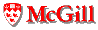# Program Size and Structure Metrics

Last updated: December 9, 2002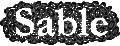Dynamic metrics for program size and structure try to answer the question: how large is a program and how complex is its control structure?

## Program Size Metrics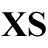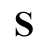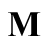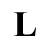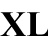Before dynamic loading became commonplace, an approximation of this metric was commonly provided by the size of the executable file. With dynamic loading in place, the program has to be run to obtain a useful measurement of its size. We propose three metrics to characterize a program's run time size, which are progressively more dynamic.
• size.load.value: The number of byte code instructions loaded. Whenever a class is loaded, its size in byte code instructions is added to a running total. The standard libraries are excluded from this measurement, since they distort the numbers (`java.*`, `javax.*`, `sun.*`, `com.sun.*`). This is the closest equivalent to the static size of an executable.
• size.run.value: The number of byte code instructions touched. This metric sums the total number of byte code instructions which are executed at least once in the entire duration of the program s execution. Run size is smaller than load size, since dead code is not counted.
• size.hot.percentile: The number of byte code instructions responsible for 90% of execution. This metric is a percentile, obtained by counting the number of times each byte code instruction is executed, sorting the instructions by frequency, and reporting the number of (most frequent) byte codes which represent 90% of executed byte codes. Hot size is smaller than load size, and we expect it to be the most robust with respect to program input.
Of these three metrics we consider size.run.value the most important in characterizing program size. It is unambiguous, not influenced by dead code, robust and machine-independent. To further simplify, size.run.value can be mapped to a classification in five categories: XS,S,M,L,XL. A program printing "hello world", with 4 byte codes touched, is in the XS category, mtrt, with 7793 byte codes is in the M category, while javac with 23K byte codes is in the L category.

## Program Structure Metrics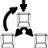The following metrics characterize the complexity of program structure by measuring instructions that change control flow (if, switch, invokeVirtual). A program with a single large loop is considered simple, as opposed to a program with multiple loops and/or many control flow changes within a singe loop.
• structure.controlDensity.value: The total number of control byte codes touched divided by the total number of byte codes touched.
• structure.changingControlDensity.value: The total number of control byte codes that change direction at least once divided by the total number of byte codes touched. This measurement is smaller than the previous one, since many control byte codes never change direction.
• structure.changingControlRate.value: The number of changes in direction divided by the number of control instruction executions. This is the most dynamic measurement. It is the equivalent of the miss rate of the simplest dynamic hardware branch predictor which predicts that a branch will follow the same direction as the last time it was executed. The metric assumes that the branch history table, as this prediction scheme is known, is free from interference or capacity misses.
Of these metrics, structure.ControlDensity.value characterizes best the complexity of program structure. It is the reciprocal of average basic block size.